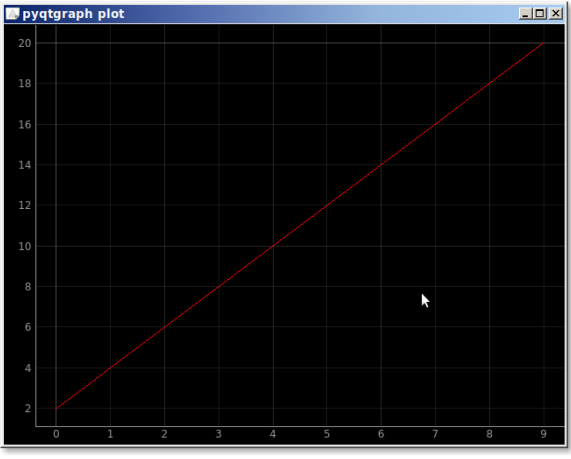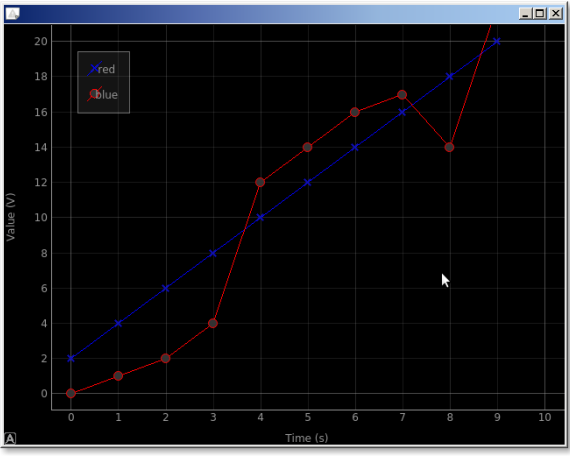A simple plot can be created with the module pyqtgraph. Mind you, it’s one of the libraries for plotting, there are others like matplotlib.

We start with importing pyqtgraph and defing the plotting data (x and y). Then we plot the data using pg.plot().Related course:
Create PyQt Desktop Appications with Python (GUI)

## pyqtgraph plot

The example below creates a plot using pyqtgraph.

We can set additional properties of the chart like the labels, add a legend, the colors.
The output will look like this:In code its like this: